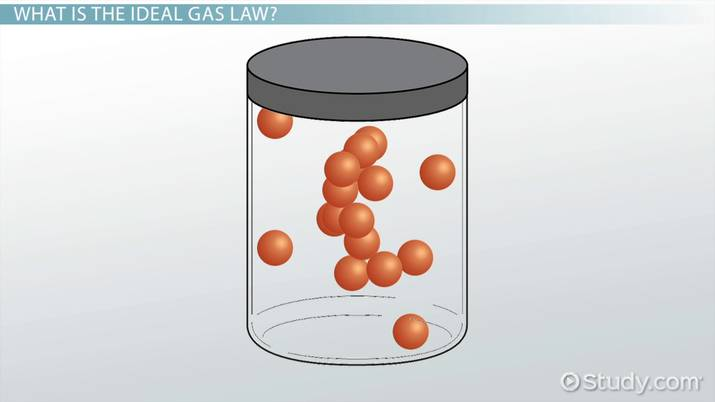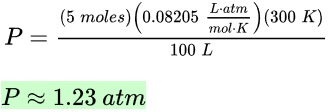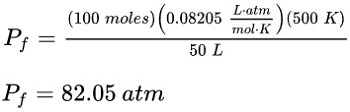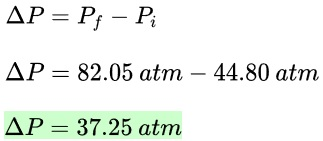# Ideal Gas Law Problems & Solutions

Lesson Transcript
Instructor: Matthew Bergstresser

Matthew has a Master of Arts degree in Physics Education. He has taught high school chemistry and physics for 14 years.

The ideal gas law describes the relationship between the pressure, volume, temperature, and number of moles of an ideal gas. Discover the ideal gas law equation and explore sample problems and solutions involving the ideal gas law. Updated: 01/20/2022

## What Is the Ideal Gas Law?

Breathing is something our bodies have been doing since we were born. We can calculate the pressure, volume, temperature, and amount of gas we inhale and exhale. Before we do a calculation involving breathing, let's learn more about the ideal gas law.

First off, what is an ideal gas? In physics and chemistry, scientists derive equations for perfect-case scenarios because, it many cases, situations are so close to ideal that we can assume they are ideal. An ideal gas is a gas where the gas particles are in a giant container bouncing into and off each other when they collide, like microscopic billiard balls. Also, the gas's temperature and kinetic energy (energy of motion) are proportional.

The ideal gas law relates pressure, volume, the molar amount of the gas, and its temperature. It's given by the equation:

PV = nRT, where

• P is pressure of the gas in atmospheres (atm).
• V is volume of the gas in liters (L).
• n is the amount of gas in moles.
• R is the ideal gas constant 0.08205 liter-atmospheres-per-mole-Kelvin (L⋅atm / mole⋅K)
• T is the temperature of the gas in Kelvin (K).

Let's go through some examples using the ideal gas law.An error occurred trying to load this video.

Try refreshing the page, or contact customer support.

Coming up next: Dalton's Law of Partial Pressures: Calculating Partial & Total Pressures

### You're on a roll. Keep up the good work!

Replay
Your next lesson will play in 10 seconds
• 0:00 What Is the Ideal Gas Law?
• 1:34 Example 1
• 2:44 Example 2
• 4:41 Example 3
• 6:10 Example 4
• 7:44 Lesson Summary
Save Save

Want to watch this again later?

Timeline
Autoplay
Autoplay
Speed Speed

## Example 1

5 moles of nitrogen gas is in a 100 liter fixed cylinder at 300 Kelvin. What is the pressure of the gas?

It's often a good idea to rearrange equations to solve for the variable being asked about. In this case, we need to get the equation in the form of P = . . . We'll do that after we organize the values we'll need.

• P = ?
• V = 100 L
• n = 5 moles
• R = 0.08205 L⋅atm / mole⋅K
• T = 300 K

Alright, so let's begin with formula PV = nRT and change that appropriately so it now is P = nRT/V. Now we can plug in the values to determine the pressure. We get:Simple!

## Example 2

A 50 L sealed cylinder contains 100 moles of helium gas. Its pressure at 273 K is 44.80 atm. What is the change in pressure when the gas is heated to 500 K?

This problem is a ''before and after'' scenario. We need the change in pressure (ΔP), which is the initial pressure subtracted from the final pressure (Pf - Pi). As before, we'll organize the values we'll need.

• Δ P = ?
• Pf = ?
• Pi = 44.80 atm
• V = 50 L constant
• n = 100 moles constant
• R = 0.08205 L⋅atm / mole⋅K
• Ti = 273 K
• Tf = 500 KNotice that we don't have initial and final subscripts for the molar amount of gas and volume. This is because the number of moles of gas doesn't change. The volume of the gas can't change either because it's a sealed cylinder. The only things that can change are the temperature and pressure. Plugging in the numbers is next and we get:## Example 3

25 moles of oxygen gas are in a cylinder with a piston that is stuck at a volume of 0.5 L. Initially the pressure inside the cylinder is 3.2 atm, and the temperature is 400 K. To what temperature does the gas have to be heated for the pressure to reach 4 atm?

To unlock this lesson you must be a Study.com Member.

### Register to view this lesson

Are you a student or a teacher?